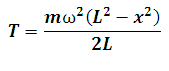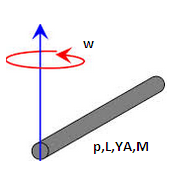# Tension in a rotating rod fixed at one end Calculator

This Calctown Calculator calculates the tension from the free end in a rod rotating about one fixed end.

kg
rad/s
m
m

#### Result

NClick here to view image

where

T = tension at distance x from the free end

x = distance from the free end

L =  lenght of rod

m = mass of rod

ω = angular velocity of rod